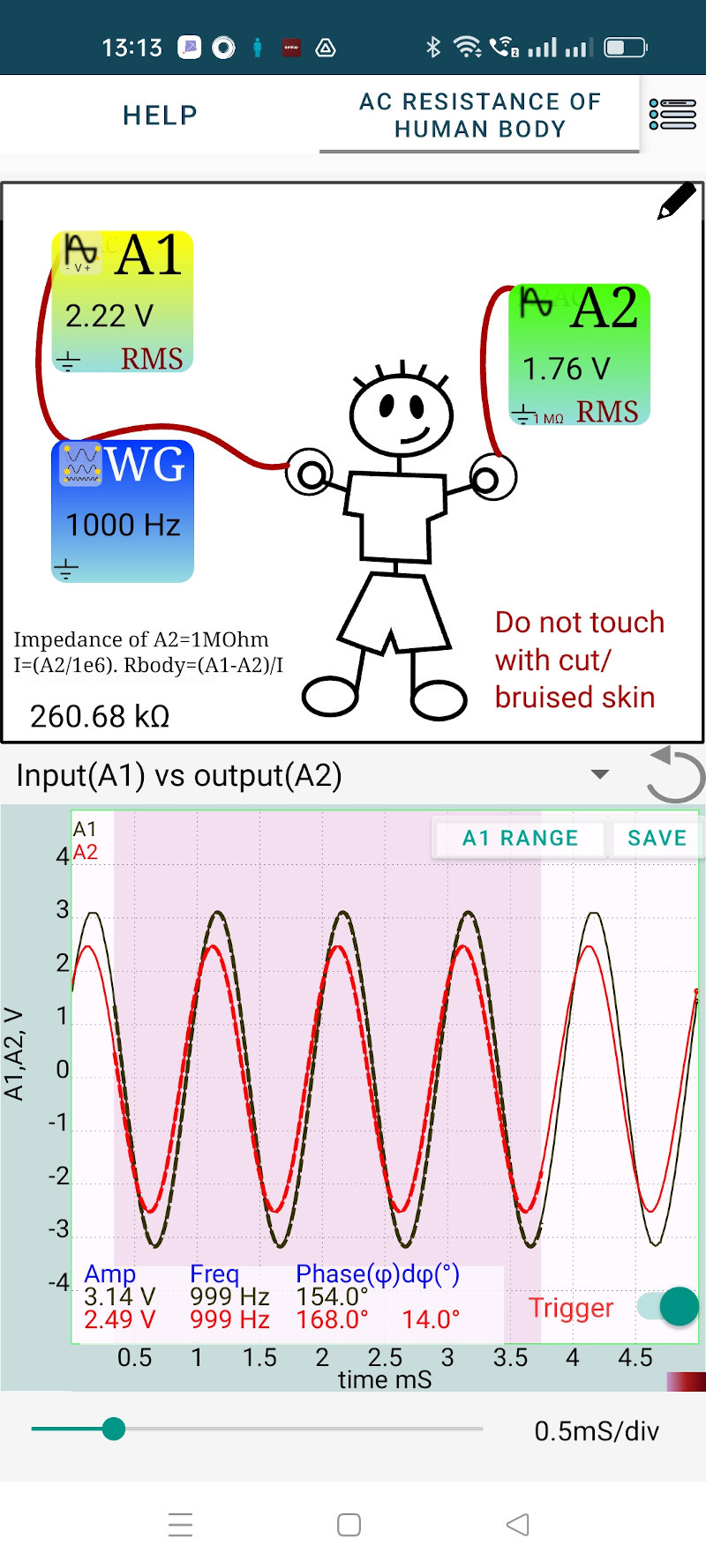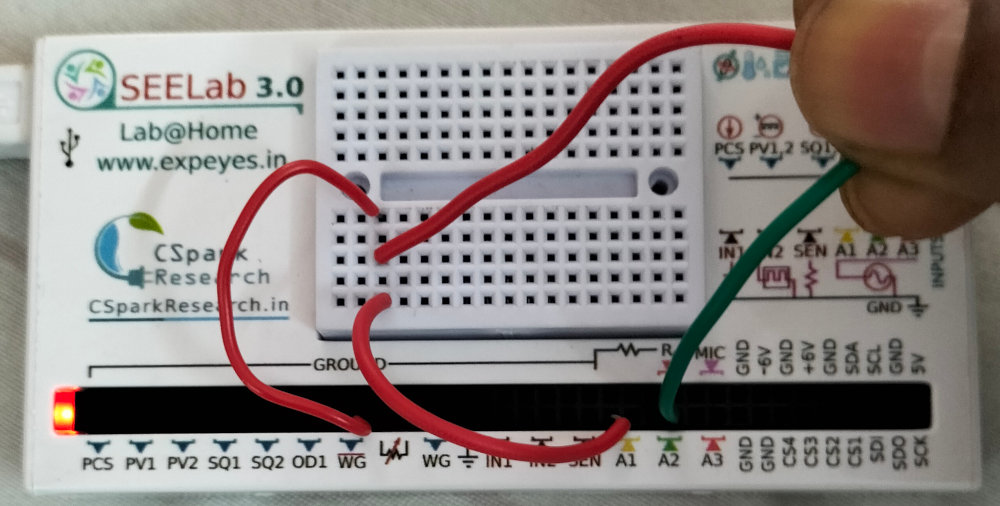# ExpEYES

## AC Resistance of Human Body

We have measured the DC resistance of human body ad the value is found to be very high. Is there any difference in the case of an AC voltage. We are using a 1000 Hz sine wave having an amplitude of 3 volts. The conenctions are shown in the figure below.

• Connect A1 to WG
• Connect one end of a wire to WG
• Connect one and of another wire to A2
• Hold the free ends of the wire
• Adjust the pressure on the wires to make the voltage at A2 more than half of that at A1.

The resistance between WG and A2 is calculated and displayed. The current from WG flows through your body to A2 and reaches ground through the input resistance of A2 (1 Mohm).

• Current into A2 = Voltage at A2 / 1 MOhm = 1.76/1e6 = 1.76 uA
• Body resistance is (2.22-1.76)/1.76e-6 = 261 kOhm### Why the AC resistance is lower

Under the same conditions the AC resistance seems to be very small. We also notice a phase difference between the traces. The AC undergoes a phase shift while passing through the body.

Phase shift means some capacitance in the ciecuit. The wire tip and blood inside our body can form a capacitor, where the skin acts as the dielectric. This guess can be further explored by sticking a foil on the hand and connecting the wire to it.

The results and the setup are shown below.